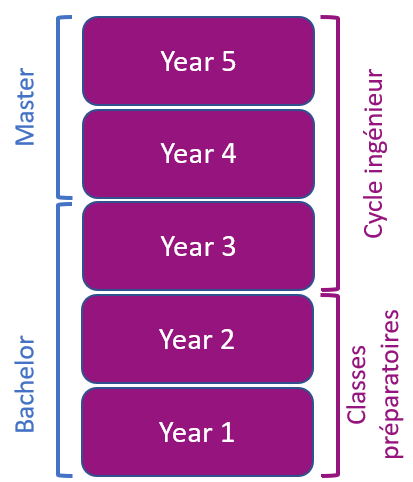# Waves and signal propagation - 3AMSC312

A+Augmenter la taille du texteA-Réduire la taille du texteImprimer le documentEnvoyer cette page par mail cet article Facebook Twitter Linked In
• #### Number of hours

• Lectures : 12.0
• Tutorials : 6.0
• Laboratory works : 9.0
• Projects : -
• Internship : -
• Written tests : -
ECTS : 2.5
• Officials : Pierre LEMAITRE AUGER

### Goals

To know how to use the basic concepts related to waves: propagation, reflection / refraction, energy attenuation. Being able to calculate the phase and amplitude of plane and spherical waves. Being able to calculate the phase and group velocities and of an electromagnetic wave in a given medium.

To know how to use the concepts related to transmission lines. Being able to analyze a transmission line in the frequency domain and in the temporal domain.

To know the concept of modes in an optical fiber. Being able to read the data sheets of an optical fiber. Being able to do a power balance of an optical link and to be able to calculate the dispersion in an optical fiber.

Content

1. Introduction
2. Electromagnetic waves
3. * Electromagnetic quantities, Maxwell's equations, constitutive equations, wave equation, general solution, properties of the electromagnetic plane wave in an homogeneous media, reflection / transmission at the limit of two dielectrics, Fresnel coefficients, TE and TM polarizations, waves in a metal, phase velocity, group velocity, normal and abnormal dispersions.
4. Transmission Lines
5. # Frequency Domain
6. # * Complex notation, lossless lines, lines ended with a complex impedance, current, voltage, local impedance, reflection coefficient, transmission coefficient, TOS
7. # Time Domain
8. # * Usefull parameters in the time domain, propagation time, reflection coefficients, analytical model, multiple reflections
9. Fiber Optics
10. * Physical principle (ray theory), description of an optical fiber, fiber types: step-index or graded index, LP modes of a step index fiber, Helmholtz equation and its solutions, the dispersion relation, b-V curves, chromatic and modal dispersions, bandwidth and losses

Prerequisites

Knowledge of electromagnetism
Knowledge of vectorial calculus (scalar and vector products, gradient, divergence, Rotational ...)
Basic knowledge of analogical electronics

Tests

1. Exam (Exam1): 1.5 hour with documentation and calculator
3. Practical Work (TP): graded activity
• Re-sit Exam (Exam2): new grade replaces all previous ones

Calendar

The course exists in the following branches:

see the course schedule for 2022-2023

Course ID : 3AMSC312
Course language(s):The course is attached to the following structures:

You can find this course among all other courses.

Bibliography

1. W. C. Elmore and M. A. Heald, Physics of Waves: Dover Publications, 1969.
2. D. M. Pozar, Microwave Engineering: Wiley, 2011.
3. B. E. A. Saleh and M. C. Teich, Fundamentals of photonics: Wiley-Interscience, 2007.

A+Augmenter la taille du texteA-Réduire la taille du texteImprimer le documentEnvoyer cette page par mail cet article Facebook Twitter Linked In

Date of update June 25, 2015

## French engineering curriculum